X
Galloping Stirrups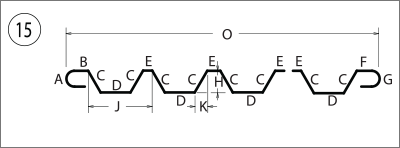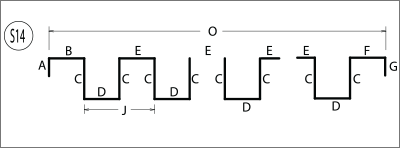* Shape 15 and S14 Complete and Partial Explanation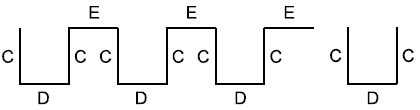A complete set consists of C, D, C, and E dimensions. The example contains 3 complete sets (CMP=3).

Partial can be 0, 1, 2, or 3 legs in the last (incomplete) set. Partials typically consist of a C, D, and C dimension, like  in the example. (PTL=3)

Spirals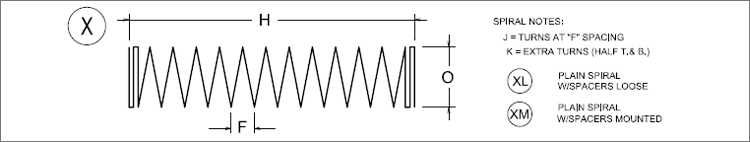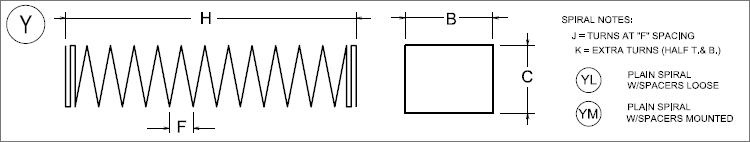Notes
1. aSa Typical Bar Bends include only shapes 1-32, T1-T17, S1-S15, and X, XL, XM, Y, YL, and YM.
2. All dimensions are out-to-out of bar except A and G on standard 180 and 135 degree hooks.
3. J dimension on 180 degree hooks to be shown only where necessary to restrict hook size; otherwise, standard hooks are to be used.
4. Where J is not shown, J will be kept equal to or less than H on those bars. Where J can exceed H, it should be shown.
5. H dimension stirrups to be shown where necessary to fit within concrete.
6. Unless otherwise noted diameter (DIA) D is the same for all bends and hooks on a bar.
7. Where slope differs from 45 degree dimensions, H and K must be shown.
8. Where bars are to be bent more accurately than standard bending tolerances, bending dimensions which require closer fabrication should have limits indicated.
9. Figures in circles show types (shapes).
10. For recommended diameter (DIA) D of bends, hooks, etc., see CRSI or ACI tables.
11. Shapes S1-S15, T1-T17 apply to bar sizes #3 through #8.
12. J dimension on shape T14, T16 is assumed to be equal to K if not specified.Light Bending – All #3 and all stirrups, column ties, and #4 through #8 bars that are bent > 6 points; bent > 1 plane; radius bent with >1 radius in any one bar, or a combination of radius and other bending (radius bending being defined as all bends heaving a radius of 12” or more to inside of bar).

Heavy Bending - #4 through #18 bars that are bent < 6 points, radius bent to 1 radius, and bending not otherwise defined.

Source